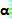Friday, December 12, 2008

Irodov Problem 1.256There six forces acting on the cylinder, i) the two normal reaction from each of the planks of N each, ii) the two forces of friction f between the planks and the cylinder surface that resists any slipping, iii) the force F with which the cylinder is being pulled downwards and iv) the force of gravity mg acting downwards. If the acceleration of the cylinder is a then, applying Newton's laws in the vertical and horizontal directions we have,The forces of normal reaction and that due to gravity pass through the axis of rotation of the cylinder. The torque on the cylinder is generated by three forces that act tangentially on the cylinder's surface at a distance r (the radius of the cylinder) from the axis, i) the force F and ii) the two forces of friction f each from each of the planks. The torque due to the forces of friction is opposed to that due to F. The moment of intertia of the cylinder about its axis of rotation is. Assuming that the cylinder's angular acceleration iswe have,If there is no slipping between the cylinder and the planks then,From (4) and (3) we have,From (5) and (2) we have,The maximum friction force f that the planks can offer is Nk from each of the planks, in other words,